# ISEE Middle Level Math : How to find the area of a rectangle

## Example Questions

### Example Question #31 : How To Find The Area Of A Rectangle

Use the following to answer the question.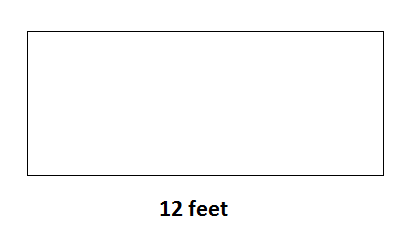Find the area of the rectangle if it's width is half of it's length.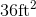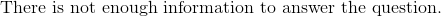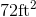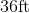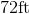Explanation:

To find the area of a rectangle, we use the following formula: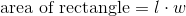where l is the length and w is the width of the rectangle.

Now, given the rectangle,we can see the length is 12 feet.  We also know the width is half of the length.  Therefore, the width is 6 feet.  Knowing this, we can substitute into the formula.  We get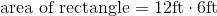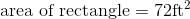### Example Question #31 : Rectangles

The Smartboard in your math classroom is 3 feet tall and 20 feet long. What is the area of the surface of the Smartboard?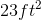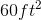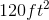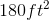Explanation:

The Smartboard in your math classroom is 3 feet tall and 20 feet long. What is the area of the surface of the Smartboard?

This problem gives us a rectangle and asks us to find the area.

The area of a triangle can be found by the following formula.Where l and w are length and width, respectively.

Plug in our length and width and solve.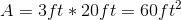So, we get 60 ft squared for our answer.

### Example Question #32 : Rectangles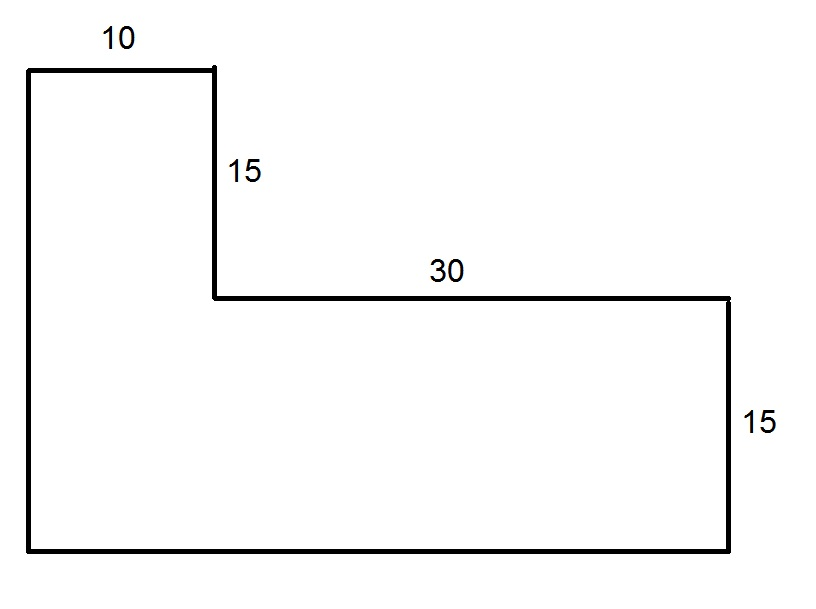In the above figure, all angles are right angles.

Give the area of the figure.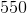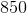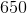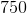Explanation:

Construct an additional segment as shown below.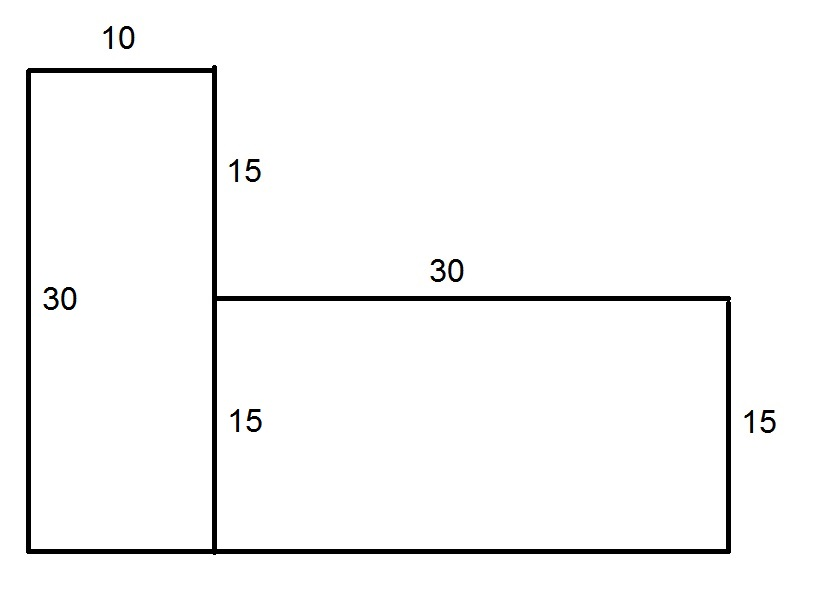Note that the figure can be divided into two rectangles. Also note that, since opposite sides of a rectangle are of the same length, we can fill in some sidelengths, as noted above.

The two rectangles each have areas that are the product of their lengths and widths: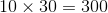and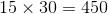Add these areas: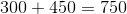, the area of the shape.

### Example Question #32 : Rectangles

You are designing a book cover for a local artist. If the cover will be made from one piece of material that is 14 inches by 8 inches, how much area will the book cover take up?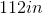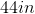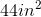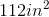Explanation:

You are designing a book cover for a local artist. If the cover will be made from one piece of material that is 14 inches by 8 inches, how much area will the book cover take up?

We are asked to find the area of a rectangle here.

To find the area of a rectangle simply use the following: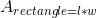So, plug in our length and width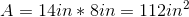So our answer is 112 inches squared.

### Example Question #35 : How To Find The Area Of A Rectangle

A rectangular postage stamp has a width of 3 cm and a height of 12 cm. Find the area of the stamp.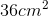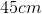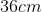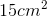Explanation:

A rectangular postage stamp has a width of 3 cm and a height of 12 cm. Find the area of the stamp.

To find the area of  a rectangle, we must perform the following: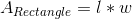Where l and w are our length and width.

This means we need to multiply the given measurements. Be sure to use the right units!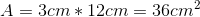And we have our answer. It must be centimeters squared, because we are dealing with area.

### Example Question #31 : Rectangles

Find the area of a rectangle that has a length of 8 cm and a width that is half the length.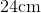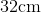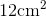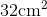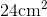Explanation:

To find the area of a rectangle, we will use the following formula:where l is the length and w is the width of the rectangle.

Now, we know the length of the rectangle is 8cm.  We also know the width is half the length.  Therefore, the width is 4cm.  Knowing this, we can substitute into the formula.  We get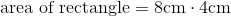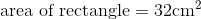### Example Question #32 : Rectangles

Find the area of a rectangle with a width of 7cm and a length that is 2 times the width.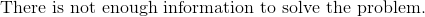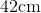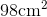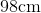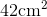Explanation:

To find the area of a rectangle, we will use the following formula:where l is the length and w is the width of the rectangle.

Now, we know the rectangle has a width of 7cm.  We also know the length is 2 times the width.  Therefore, the length is 14cm.  Knowing this, we can substitute into the formula.  We get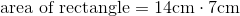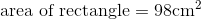### Example Question #2021 : Isee Middle Level (Grades 7 8) Mathematics Achievement

Find the area of a rectangle with a length of 8cm and a width that is half the length.Explanation:

To find the area of a rectangle, we will use the following formula:where l is the length and w is the width of the rectangle.

Now, we know the length of the rectangle is 8cm.  We also know the width of the rectangle is half of the length.  Therefore the width is 4cm.  Knowing this, we can substitute into the formula.  We get### Example Question #191 : Plane Geometry

Find the area of a rectangle with a width of 3in and a length that is three times the width.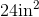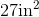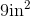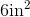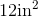Explanation:

To find the area of a rectangle, we will use the following formula:where l is the length and w is the width of the rectangle.

Now, we know the width of the rectangle is 3in.  We also know the length is 3 times the width.  Therefore, the length is 9in.  Knowing this, we can substitute into the formula.  We get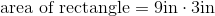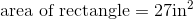### Example Question #31 : Rectangles

Find the area of a rectangle with a length of 14in and a width that is half the length.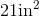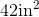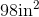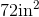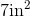Explanation:

To find the area of a rectangle, we will use the following formula:where l is the length and w is the width of the rectangle.

Now, we know the length of the rectangle is 14in.  We also know the width of the rectangle is half the length.  Therefore, the width is 7in.

Knowing this, we will substitute into the formula.  We get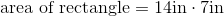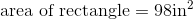### All ISEE Middle Level Math Resources